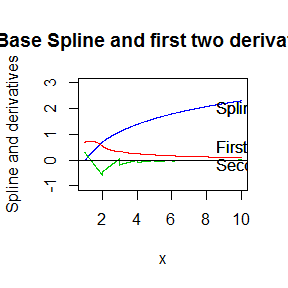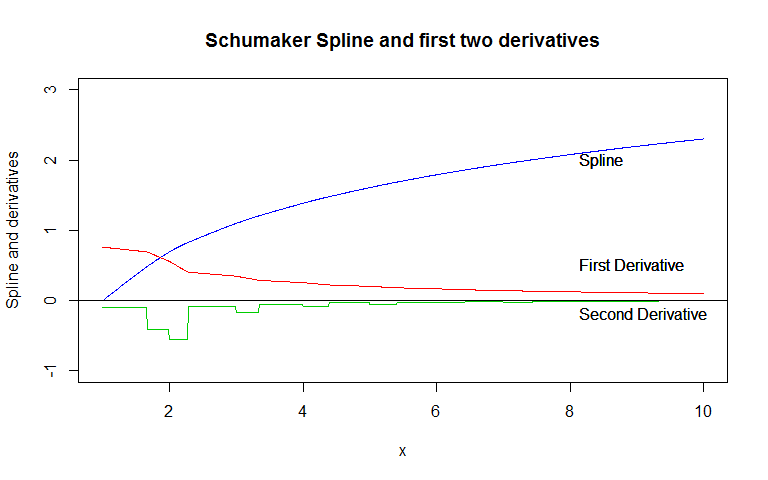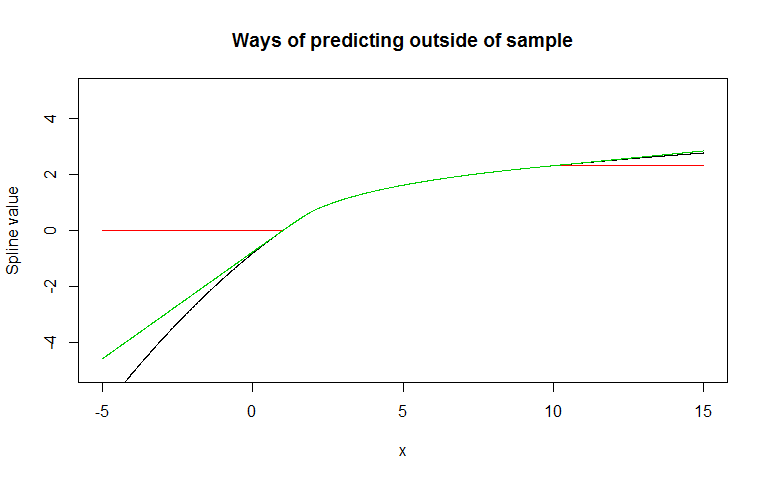This package implements the schumaker spline for one dimensional interpolation. This is the first publicly available R package to give a shape-constrained spline without any optimisation being necessary. It also has significant speed advantages compared to the other shape constrained splines. It is intended for use in dynammic programing problems and in other cases where a simple shape constrained spline is useful.

This package first illistrates that the base splines can deliver nonconcave splines from concave data. It follows by describing the options the schumaker spline provides. It then describes a consumption smoothing problem before illustrating why the schumaker spline performs significantly better than existing splines.

Finally the speed of this spline in comparison with other splines is described.

## The schumaker spline and base splines

We can show that the base splines are not shape preserving. Now we can plot the monotonic spline (you can experiment with the other ones for a similar result):

x = seq(1,10)
y = log(x)

xarray = seq(1,10,0.01)

BaseSpline = splinefun(x,y, method = "monoH.FC")
Base0 = BaseSpline(xarray)
Base1 = DerivBaseSpline(xarray)
Base2 = Deriv2BaseSpline(xarray)

plot(xarray, Base0, type = "l", col = 4, ylim = c(-1,3), main = "Base Spline and first two derivatives",
ylab = "Spline and derivatives", xlab = "x")
lines(xarray, Base1, col = 2)
lines(xarray, Base2, col = 3)
abline(h = 0, col = 1)
text(x=rep(8,8,8), y=c(2, 0.5,-0.2), pos=4, labels=c('Spline', 'First Derivative', 'Second Derivative'))Here you can see that the first derivative is always positive - the spline is monotonic. However the second derivative moves above and below 0. The spline is not globally concave.

Now we can show the schumaker spline:

library(schumaker)
SchumSpline = schumaker::Schumaker(x,y)
Schum0 = SchumSpline$Spline(xarray) Schum1 = SchumSpline$DerivativeSpline(xarray)
Schum2 = SchumSpline$SecondDerivativeSpline(xarray) plot(xarray, Schum0, type = "l", col = 4, ylim = c(-1,3), main = "Schumaker Spline and first two derivatives", ylab = "Spline and derivatives", xlab = "x") lines(xarray, Schum1, col = 2) lines(xarray, Schum2, col = 3) abline(h = 0, col = 1) text(x=rep(8,8,8), y=c(2, 0.5,-0.2), pos=4, labels=c('Spline', 'First Derivative', 'Second Derivative'))Here the second derivative is always negative - the spline is globally concave as well as monotonic. ## Optional Settings There are three optional setting in creating a spline. Firstly the gradients at each of the (x,y) points can be input to give more accuracy. If not supplied these are estimated from the points. Secondly if Vectorised = TRUE arrays can be input to the spline. If arrays will never be input to the spline then you can set Vectorised = FALSE for a small speed improvement (about 30%). Finally there are three options for out of sample prediction. • Curve - This is where the quadriatic curve that is present in the first and last interval are used to predict points before the first interval and after the last interval respectively. • Linear - This is where a line is extended out before the first interval and after the last interval. The slope of the line is given by the derivative at the start of the first interval and end of the last interval. • Constant - This is where the first and last y values are used for prediction before the first point of the interval and after the last part of the interval respectively. The three out of sample options are shown below. Here you can see in black the curve is extended out. In green the ends are extrapolated linearly whilst red has constant extrapolation. Note that there is no difference between the 3 within sample. x = seq(1,10) y = log(x) xarray = seq(-5,15,0.01) SchumSplineCurve = Schumaker(x,y, Extrapolation = "Curve" )$Spline

SchumSplineConstant = Schumaker(x,y, Extrapolation = "Constant")$Spline SchumSplineLinear = Schumaker(x,y, Extrapolation = "Linear" )$Spline

SchumSplineCurveVals    = SchumSplineCurve(xarray)
SchumSplineConstantVals = SchumSplineConstant(xarray)
SchumSplineLinearVals   = SchumSplineLinear(xarray)

plot(xarray, SchumSplineCurveVals, type = "l", col = 1, ylim = c(-5,5),
main = "Ways of predicting outside of sample", ylab = "Spline value", xlab = "x")
lines(xarray, SchumSplineConstantVals, col = 2)
lines(xarray, SchumSplineLinearVals, col = 3)## Example: A simple consumption smoothing problem

Consider a consumer that has a budget of $$B_t$$ at time $$t$$ and a periodic income of $$1$$. They have a periodic utility function given by:

$$u_t = \epsilon_t x_t^{0.2}$$

where $$x_t$$ is spending in period $$t$$ and $$\epsilon_t$$ is the shock in period $$t$$ drawn from some stationary nonnegative shock process with pdf $$f(\epsilon)$$.

The problem for the consumer in period $$t$$ is:

$$V(B_t | \epsilon_{t}) = \max_{0 < x_t < B} \hspace{0.5cm} \epsilon_t x_t^{0.2} + \beta E_t[ V(B_{t+1})]$$

Where $$\beta$$ is a discounting factor and $$B_{t+1} = 1 + B_t - x_t$$.

### Algorithm

We can first note that due to the shock process it is not possible to get analytical equations to describe the paths of spending and the budget over the long term. We can get a numerical solution however. The key step is to find expressions for the Expected value function as a function of $$B_{t+1}$$. With this we can run simulations with random shocks to see the long term distributions of $$x_t$$ and $$B_t$$. The algorithm we will use is:

1. We discretize the budget statespace.
2. We make an initial guess of the future value function $$E[ V(B_{t+1})]$$ at every value of $$B_{t+1}$$ in our discretized statespace. A deterministic approximation of the problem (assume shocks will be $$E[\epsilon_{t}]$$ forever) is often good for this.
3. We use the schumaker spline to join together our estimates at each point in the discretized statespace into an interpolation function.
4. At every point in the statespace we create updated estimates
• We use a cubature package to estimate $$\int^\infty_{0} V(B_t | \epsilon_t ) f(\epsilon_t) d\epsilon_t$$
• In this integral we use a one dimensional optimizer along with the above equation to evaluate $$V(B_t | \epsilon_t )$$
1. Check a convergence criteria. Are the new $$V(B_t | \epsilon_t )$$ values very close to the old values?
• If they are we end the algorithm
• If they are not we go back to point 3.

This strategy relies on the consumption problem being a contraction mapping. This means that if we use this algorithm we will coverge to a fixed point function. The turboem package (squareem method) can be useful here in accelerating the convergence.

### Why a schumaker spline is necessary

There are a few reasons we need the spline to be shape preserving and without optimization to make this work:

• Shape preservation is necessary so the spline is globally concave (the $$V(B_t | \epsilon_t )$$ values will always be concave as the periodic utility function is always concave). This is necessary for the one dimensional optimisation step. The periodic utility function is concave and the future value function needs to be concave (in $$B_{t+1}$$) to guarantee a unique local optima. Other splines can incorporate convexities in the intervals between interpolation points which can lead to multiple local optima.

• There do exist shape preserving splines that incorporate an optimization step (ie scam, cobs). These are not effective for this kind of problem because it is not possible to converge to a fixed point. In each iteration the optimization settles on a slightly different parameter set which means the future value function can “jump around”. In addition the optimization step can take a significant amount of time because evaluations of the future value function spline take longer.

## Speed

• The schumaker spline is faster to create to create splines and predict with splines than the other constrained splines packages (cobs and scam) by a large amount.
• The schumaker spline takes about 190 times longer to create than the base spline.
• The schumaker spline takes about half as long to predict an array of points than the base spline.
• The schumaker spline takes about 25% as long to predict a single point than the base spline.

These microbenchmarks are available below:

library(microbenchmark)
library(cobs)
library(scam)
## Loading required package: mgcv
## This is mgcv 1.8-7. For overview type 'help("mgcv-package")'.
## This is scam 1.1-9.
x = seq(1,10)
y = log(x)
dat = data.frame(x = x, y = y)
xarray = seq(0,15,0.01)

microbenchmark(
Schumaker(x,y),
splinefun(x,y,"monoH.FC"),
scam(y~s(x,k=4,bs="mdcx",m=1),data=dat),
cobs(x , y, constraint = c("decrease", "convex"), print.mesg = FALSE),
unit = "relative"
)
## Unit: relative
##                                                                  expr
##                                                       Schumaker(x, y)
##                                           splinefun(x, y, "monoH.FC")
##                 scam(y ~ s(x, k = 4, bs = "mdcx", m = 1), data = dat)
##  cobs(x, y, constraint = c("decrease", "convex"), print.mesg = FALSE)
##       min       lq     mean   median       uq      max neval
##  280.0897 201.8949 196.7173 181.7697 188.8911 177.3236   100
##    1.0000   1.0000   1.0000   1.0000   1.0000   1.0000   100
##  390.2860 285.5328 279.4938 253.6104 273.4937 275.0180   100
##  661.1598 490.4575 479.8921 430.6222 459.3527 723.2516   100
BaseSp =   splinefun(x,y,"monoH.FC")
SchuSp =   Schumaker(x,y)$Spline ScamSp = scam(y~s(x,k=4,bs="mdcx",m=1),data=dat) CobsSp = cobs(x , y, constraint = c("decrease", "convex"), print.mesg = FALSE) ScamPr = function(x){ predict.scam(ScamSp,data.frame(x = x))} CobsPr = function(x){ predict(CobsSp, x)[,2] } microbenchmark( SchuSp(xarray), BaseSp(xarray), ScamPr(xarray), CobsPr(xarray), unit = "relative" ) ## Unit: relative ## expr min lq mean median uq ## SchuSp(xarray) 1.000000 1.000000 1.000000 1.000000 1.000000 ## BaseSp(xarray) 1.789826 1.806502 1.445776 1.811464 1.760317 ## ScamPr(xarray) 41.420161 48.773831 35.122166 53.518808 44.886020 ## CobsPr(xarray) 1.538982 1.600003 1.537026 1.684772 1.529704 ## max neval ## 1.000000 100 ## 1.021225 100 ## 11.617287 100 ## 1.311298 100 SchuSp = Schumaker(x,y, Vectorise = FALSE)$Spline
microbenchmark(
SchuSp(runif(1)),
BaseSp(runif(1)),
ScamPr(runif(1)),
CobsPr(runif(1)),
unit = "relative"
)
## Unit: relative
##              expr        min         lq       mean     median         uq
##  SchuSp(runif(1))   1.000000   1.000000   1.000000   1.000000   1.000000
##  BaseSp(runif(1))   5.499869   4.888792   3.491205   4.144650   4.235326
##  ScamPr(runif(1)) 215.444284 171.513992 127.834016 159.824746 147.774414
##  CobsPr(runif(1))  10.249803   9.036891   6.021769   7.355108   6.872559
##         max neval
##   1.0000000   100
##   0.5362518   100
##  21.1477504   100
##   0.9316023   100

## Reference

The key reference for the schumaker spline is Judd’s Numerical Methods in Economics (1998). This presents precisely how to create the spline and it is simple to reconcile the code with the equations from this book. This book also gives more detail on solving dynammic control problems. A further reference which advocates the use of the schumaker spline is Ljungqvist and Sargent’s Recursive Economic Theory.